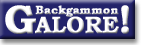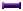Cube Handling in RacesN-roll vs n-roll bearoff

 From: Chuck Bower Address: bower@bigbang.astro.indiana.edu Date: 15 December 1997 Subject: Re: Trailing when leader is 2 away is a mandatory double if ... Forum: rec.games.backgammon Google: 673qit\$b7t\$1@dismay.ucs.indiana.edu

```Alexander Nitschke wrote:
> One additional comment: In money game 15 checkers each on the ace point
> is an initial double. So the trailer must be more reluctant to double
> than in money game.

I believe you, but this surprises me.  I thought the "rule" was to wait
until it's five roll vs. five roll for the initial double, and four roll
vs. four roll for the redouble.  Could someone please elaborate?
```

 Sam Pottle  writes: ```Actually, Kleinman discusses this in _Vision Laughs At Counting_, so it's not exactly new theory. Until I read Kleinman I thought as you did, probably because Robertie's treatment in _Advanced Backgammon_ only went up to the 5-roll v. 5-roll position. Robertie never actually says, though, that the 5 roll position is the earliest initial double. How should we approach the double/no-double decision in a pure 8-roll v. 8-roll position? Well, first of all, we're a solid favorite (about 67% cubeless), so an initial double is at least worth thinking about. We lose our market whenever we roll doubles and our opponent rolls non-doubles (about 14% of the time). These are big market losers, too -- our winning chances in the resulting 6-roll v. 7-roll position are over 90%. Average sequences (both players roll doubles, or both roll non-doubles) wash; the resulting 6-roll v 6-roll or 7-roll v. 7-roll positions are still double/take. What about our poor sequences? We'll be sorry we doubled whenever we roll non-doubles, opponent rolls doubles, and we roll non-doubles (about 11.5%). Opponent will then be on roll in a 6-roll v. 6-roll position, leaving her with a strong advantage, but still a roll or two away from a recube. In fact, if we've doubled, opponent will hold the 2-cube, while if we haven't, opponent will give us an initial double (which we'll take), so that we will be holding a 2-cube. So in this variation, having doubled costs us precisely the value of cube ownership of a 2-cube in a 6-roll v. 6-roll position. How much is that cube ownership worth? One clue that it's pretty valuable is the fact that opponent shouldn't redouble before reaching a 4-roll v. 4-roll position, while she has a correct initial double already. Also, that recube is pretty efficient when it comes, and it frequently does come. So, which costs more, the big market loser, or the big loss of cube ownership? Let's assume that they're about the same. Then the fact that our bad sequences are only 5/6 as numerous as our good ones tips the balance in favor of doubling. Let's put some numbers to these notions. Our equity after a good sequence is about .80 (1.60 on a 2-cube), so losing our market costs us about .60 when it happens. Our equity with opponent on roll in a 6-roll v. 6-roll position is about -.30 (-.60 on a 2-cube) if we own the cube, and about -.65 (-1.30) if opponent owns the cube. So having doubled costs us about .70 in these variations. Since .60 is bigger than 5/6 of .70, doubling turns out to be correct by a small margin. The margin is indeed quite small, as David Montgomery pointed out: .452 if you double, .450 if you don't. But the double is technically correct. Bonus questions: (Assume we're allowed more than 15 checkers per side.) -- What is the smallest number n for which an n-roll v. n-roll position is not a proper initial double? -- What is the smallest number n for which an n-roll v. (n+1)-roll position is a take? ```

 Steven Turner  writes: ```> What is the smallest number n for which an n-roll v. n-roll > position is not a proper initial double? It's n = 9 (the smallest n which can't occur in real backgammon). 33 v. 33 is a beaver. > What is the smallest number n for which an n-roll v. (n+1)-roll > position is a take? n = 18. 25 v. 26 is an initial double but not a redouble. 30 v. 31 isn't even an initial double. 193 v. 194 is a beaver. Of course anyone who plays backgammon with 387 chequers per side deserves everything they get. ```

 Jason Lee  writes: ```193 rolls vs. 194 rolls is a beaver. What seems counterintuitive at first does make sense, I think ... in such a looooooooong race, ownership of the cube is extra valuable, since over that long period, you're likely to get a pretty efficient cube at some point. Do I have that right? JLee ```

 Sam Pottle  writes: ```Yes. A 200 turn race is much closer to the continuous model of backgammon than any normal position. In the continuous model, you can beaver with cubeless chances of 40% (halfway between zero and the 80% cashpoint). With 194 vs. 193 rolls, the beavering side has a little under 41%, and the race is so "smooth" that this is enough. ```

 Tom  writes: ```Enjoyed this immensely, but can you provide some insight as to the methodology you used to arrive at these figures, ie. how did you come up with the numbers? ```

 Stephen Turner  writes: ```I just wrote a little program to calculate them: #include #define N (500) int main(){ int i, j, t; double eq1[N][N], eq2[N][N], eq0[N][N]; for (t = 0; t < N; t++) { for (i = 0; i <= t; i++) { j = t - i; if (j == 0) { eq0[i][j] = -1.0; eq1[i][j] = -1.0; eq2[i][j] = -1.0; } else if (i <= 1) { eq0[i][j] = 1.0; eq1[i][j] = 1.0; eq2[i][j] = 1.0; } else { eq0[i][j] = -5 * eq0[j][i - 1] / 6 - eq0[j][i - 2] / 6; eq1[i][j] = -5 * eq2[j][i - 1] / 6 - eq2[j][i - 2] / 6; eq2[i][j] = -5 * eq1[j][i - 1] / 6 - eq1[j][i - 2] / 6; } if (2 * eq2[i][j] > eq1[i][j]) { if (2 * eq2[i][j] > 1.0) { printf("%3d%4d [%7.4f:%7.4f:%7.4f] redouble/drop\n", i, j, eq0[i][j], eq1[i][j], eq2[i][j]); eq1[i][j] = 1.0; eq0[i][j] = 1.0; } else { printf("%3d%4d [%7.4f:%7.4f:%7.4f] redouble/take\n", i, j, eq0[i][j], eq1[i][j], eq2[i][j]); eq1[i][j] = 2 * eq2[i][j]; eq0[i][j] = 2 * eq2[i][j]; } } else if (2 * eq2[i][j] > eq0[i][j]) { printf("%3d%4d [%7.4f:%7.4f:%7.4f] initial double/take\n", i, j, eq0[i][j], eq1[i][j], eq2[i][j]); eq0[i][j] = 2 * eq2[i][j]; } else if (eq2[i][j] >= 0) printf("%3d%4d [%7.4f:%7.4f:%7.4f] no double/take\n", i, j, eq0[i][j], eq1[i][j], eq2[i][j]); else printf("%3d%4d [%7.4f:%7.4f:%7.4f] no double/beaver\n", i, j, eq0[i][j], eq1[i][j], eq2[i][j]); } printf("\n"); } return(0); } ```

### Cube Handling in Races

Bower's modified Thorp count  (Chuck Bower, July 1997)
Calculating winning chances  (Raccoon, Jan 2007)
Calculating winning chances  (OpenWheel+, Nov 2005)Doubling formulas  (Michael J. Zehr, Jan 1995)
Doubling in a long race  (Brian Sheppard, Feb 1998)
EPC example: stack and straggler  (neilkaz+, Jan 2009)
EPC examples: stack and straggler  (Carlo Melzi+, Dec 2008)
Effective pipcount  (Douglas Zare, Sept 2003)Effective pipcount and type of position  (Douglas Zare, Jan 2004)Kleinman count  (Øystein Johansen+, Feb 2001)
Kleinman count  (André Nicoulin, Sept 1998)
Kleinman count  (Chuck Bower, Mar 1998)Lamford's race forumla  (Michael Schell, Aug 2001)
N-roll vs n-roll bearoff  (David Rubin+, July 2008)
N-roll vs n-roll bearoff  (Gregg Cattanach, Nov 2002)
N-roll vs n-roll bearoff  (Chuck Bower+, Dec 1997)
Near end of game  (Daniel Murphy, Mar 1997)
Near end of game  (David Montgomery, Feb 1997)
Near end of game  (Ron Karr, Feb 1997)
One checker model  (Kit Woolsey+, Feb 1998)
Pip count percentage  (Jeff Mogath+, Feb 2001)
Pip-count formulas  (Tom Keith+, June 2004)Thorp count  (Chuck Bower, Jan 1997)
Thorp count  (Simon Woodhead, Sept 1991)
Thorp count questions  (Chuck Bower, Sept 1999)
Value of a pip  (Tom Keith, June 2004)
Ward's racing formula  (Marty Storer, Jan 1992)
What's your favorite formula?  (Timothy Chow+, Aug 2012)From GammOnLineLong messageRecommended readingRecent addition

 Book Suggestions Books Cheating Chouettes Computer Dice Cube Handling Cube Handling in Races Equipment Etiquette Extreme Gammon Fun and frustration GNU Backgammon History Jellyfish Learning Luck versus Skill Magazines & E-zines Match Archives Match Equities Match Play Match Play at 2-away/2-away Miscellaneous Opening Rolls Pip Counting Play Sites Probability and Statistics Programming Propositions Puzzles Ratings Rollouts Rules Rulings Snowie Software Source Code Strategy--Backgames Strategy--Bearing Off Strategy--Checker play Terminology Theory Tournaments Uncategorized Variations